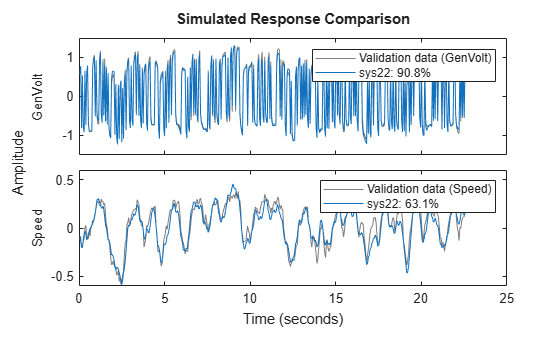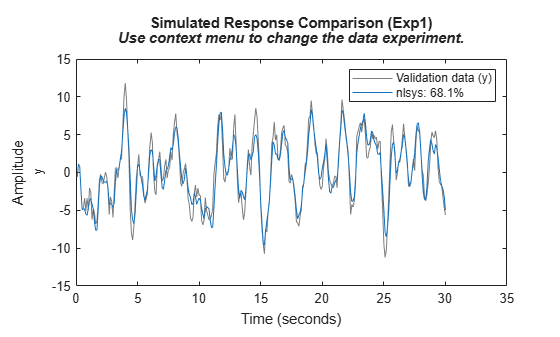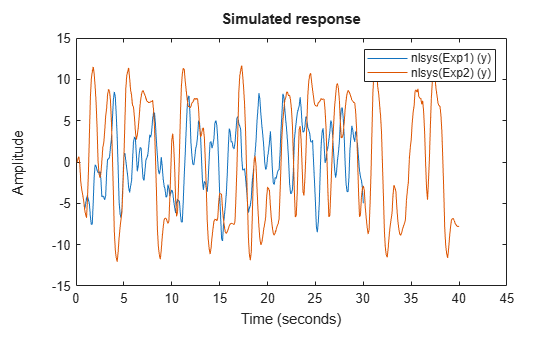# Use Timetable Data for Time-Domain System Identification

Timetables are a built-in MATLAB® datatype represented by `timetable` objects. Timetables can contain multiple variables, and are used generally to represent observations, or data values, that are ordered in time. For system identification, you can use timetable data that contains the estimation input and output signal channels. You can create timetables by using the timetable constructor, or by using the `array2timetable` command. When you import data into MATLAB from data files such as `csv` files or spreadsheets, the import process can create timetables automatically.

As a simple example, create a single-variable timetable that contains random data over a period of five seconds.

```T = seconds(1:5)'; u = rand(5,1); tt = timetable(T,u)```
```tt=5×1 timetable T u _____ _______ 1 sec 0.81472 2 sec 0.90579 3 sec 0.12699 4 sec 0.91338 5 sec 0.63236 ```

The time vector itself is not a variable. You can access the time vector `T` in `tt` by using the property` tt.Properties.RowTimes`. Other time-related properties are `tt.Properties.SampleRate` and `tt.Properties.TimeStep`.

`T = tt.Properties.RowTimes`
```T = 5x1 duration 1 sec 2 sec 3 sec 4 sec 5 sec ```

Identification typically requires uniformly sampled data. This means that the `SampleRate` and `TimeStep` properties must each contain a finite value. One exception to this requirement is the neural state-space model, which is represented by the `idNeuralStateSpace` object. To estimate the continuous-time form of this model, the timetable sample points need not be uniformly separated.

System Identification Toolbox™ supports `timetable`-based estimation data for release R2022b onwards. For earlier releases, you must use the `iddata` object to represent estimation data.

### Define Variables

In general, timetables can store nonnumeric data, as well as samples of arbitrary sizes for each time value. However, you can use only numeric scalar-valued variables to identify models. For instance, suppose that you have data for two signals `u1` and `u2` that share a common time vector `T`. There are two ways of storing these signals in a timetable:

1. As a single variable whose value is a 2-element vector `[u1(t), u2(t)]` for a given time `t`.

2. As two distinct variables, one for each signal.

In System Identification Toolbox, the second option - using distinct variables for each signal, is the only supported representation. The following example illustrates the results for both approaches.

Create a data set that contains two signals that contain random data.

```T = seconds(1:5)'; u1 = rand(5,1); u2 = rand(5,1);```

Implement option 1 by combining both `u1` and `u2` in a single variable `U`.

```tt1 = timetable(T, [u1, u2], 'VariableNames', {'U'}); head(tt1)```
``` T U _____ __________________ 1 sec 0.09754 0.15761 2 sec 0.2785 0.97059 3 sec 0.54688 0.95717 4 sec 0.95751 0.48538 5 sec 0.96489 0.80028 ```

Implement option 2 by assigning `u1` and `u2` to separate variables `u1` and `u2`.

```tt2 = timetable(T, u1, u2, 'VariableNames', {'u1','u2'}); head(tt2)```
``` T u1 u2 _____ _______ _______ 1 sec 0.09754 0.15761 2 sec 0.2785 0.97059 3 sec 0.54688 0.95717 4 sec 0.95751 0.48538 5 sec 0.96489 0.80028 ```

You cannot use `tt1` for model estimation and analysis. Only `tt2` is a valid representation.

### Estimate Model Using Specified Input and Output Channels

Estimate a model from data that contains two input channels and two output channels.

```load steamdata ttsteam head(ttsteam,4)```
``` Time Pressure MagVolt GenVolt Speed ________ ________ _______ ________ _________ 0.05 sec -1.5283 2.0584 0.57733 -0.12274 0.1 sec 1.4412 -2.005 0.75804 -0.086114 0.15 sec 1.4314 2.0584 -0.76577 -0.19845 0.2 sec 1.4412 -1.9806 0.47721 -0.20577 ```

`ttsteam` contains the input variables `Pressure` and `MagVolt` and the output variables `GenVolt` and `Speed`.

You can use any or all of the available channels, but you must be explicit about which channels to use. By default, the software interprets the last variable as a single output channel and the remaining variables as input channels.

Set the number of poles and zeros both to 2 and try to estimate a model without designating input and output channels.

```np = 2; nz = 2; sysdef = tfest(ttsteam,np,nz);```

View the default assignments for inputs and outputs.

`inputs = sysdef.InputName`
```inputs = 3x1 cell {'Pressure'} {'MagVolt' } {'GenVolt' } ```
`outputs = sysdef.OutputName`
```outputs = 1x1 cell array {'Speed'} ```
`fit = sysdef.Report.Fit.FitPercent`
```fit = 71.2432 ```

The software designates the first three variables as inputs.

Estimate a model using both input channels but only the `GenVolt` output channel.

```sys21 = tfest(ttsteam, np, nz, 'InputName',{'Pressure','MagVolt'},'OutputName','GenVolt'); outputs = sys21.Outputname```
```outputs = 1x1 cell array {'GenVolt'} ```
`fit = sys21.Report.Fit.FitPercent`
```fit = 90.8266 ```

Estimate a model using all the channels.

```sys22 = tfest(ttsteam, np, nz, 'InputName',{'Pressure','MagVolt'},'OutputName',{'GenVolt','Speed'}); fit = sys22.Report.Fit.FitPercent```
```fit = 2×1 90.8150 63.0827 ```

You can use timetables in all model validation and analysis operations that require input/output data. The estimated model stores the input and output channel information, so when you use these operations, you do not need to specify which channels to use.

`compare(ttsteam, sys22)`### Combine Data from Multiple Experiments

A single timetable can represent the data from only a single experiment. If you have multiple timetables that arise from multiple experiments, you can combine these timetables in a cell array and use this cell array for estimation and analysis. The individual timetables in the cell array are referred to as experiments. For instance, the variable `X = {tt1, tt2, tt3}` represents a multiple-experiment data set consisting of the three experiments `tt1, tt2`, and `tt3`. Each experiment must have the same data type—in this case, timetable— the same active numeric variables, and the same sample time and time units. The start time, and the number of observations in the individual experiments may be different.

Load two timetables, `tt1` and `tt2`. For the purpose of this example only, assume both timetables correspond to the same system. The timetables have different lengths, but have the same sample rate of 10 Hz.

```load sdata1 tt1 load sdata2 tt2```

Create a multiple-experiment cell array.

`MultiExpData = {tt1, tt2};`

Use `MultiExpData` to estimate a nonlinear ARX model.

```Orders = [5 5 1]; NL = idGaussianProcess; nlsys = nlarx(MultiExpData, Orders, NL);```

Compare the model response to the estimation data.

`compare(MultiExpData, nlsys);`The plot shows the fit to the first dataset. Right-click on the plot and use the context menu to pick the second data experiment to view. To access the context menu, you must generate the plot from the command window rather than from a script.

In a similar manner to the previous functions, you can perform model simulation, response prediction, and residual analysis using multiple-experiment data. For instance, simulate `nlsys` using `MultiExpData`.

`sim(nlsys,MultiExpData)`The plot displays the simulated results.